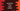# JavaScript Array every method explanation with example## JavaScript Array every method:

every method of JavaScript array is used to check if every element in an array satisfies a given callback function.

It takes one function and executes that function for each element in the array one by one. For any element, if that function returns false, every will immediately return false.

If the callback function returns true for all elements in the array, every will return true.

In this post, we will learn the syntax of every method, and how to use it with examples.

## Syntax of every method:

every method is defined as like below:

``every(function callback(item, index, array){...}, thisArg)``

Or, with arrow function:

``every((item, index, array) => {...})``

As you can see here, it takes one callback function, that takes three arguments:

item: This is the current element currently iterating by every.

index(optional): It is the index of the current element. This is an optional argument.

array(optional): It is the array where every is called. This is an optional argument.

thisArg is another optional value. If this is provided, it will be used as this while executing the function.

### Return value of every method:

every returns one boolean value.

• It returns true if the callback function returns true for all elements of the array. It will also work for any other return value that is true if we convert to Boolean.
• It returns false otherwise.

## Example of every: Check if all items in an array are even:

Let’s take an example of every to check if all items in an array are even or not:

``````function isEven(e, i, arr){
return e%2 === 0;
}

let firstArray = [1, 2, 3, 4, 5, 6];
let secondArray = [2, 4, 6, 8, 10];

console.log('For the firstArray: ',firstArray.every(isEven));
console.log('For the secondArray: ',secondArray.every(isEven));``````

Here,

• firstArray and secondArray are two arrays with different numbers.
• isEven function checks if a number is even or not. This is the callback function we are using in every.
• The first log prints the result of every with isEven for the firstArray and the second log prints the result of every with isEven for the secondArray.

If you run this program, it will give the below result:

``````For the firstArray:  false
For the secondArray:  true``````

As you can see here, for the firstArray, it returns false because all elements are not even for this array. For the secondArray, all elements are even, so it returns true.

Note that we don’t need all of these arguments in the isEven function. The second and the third arguments are optional.

``````function isEven(e){
return e%2 === 0;
}

let firstArray = [1, 2, 3, 4, 5, 6];
let secondArray = [2, 4, 6, 8, 10];

console.log('For the firstArray: ',firstArray.every(isEven));
console.log('For the secondArray: ',secondArray.every(isEven));``````

It will give a similar result.

## Example of every with an arrow function, check if all numbers are odd in an array:

We can also use every with arrow functions. Let’s change the above example to check if all numbers are odd by using an arrow function:

``````let firstArray = [1, 2, 3, 4, 5, 6];
let secondArray = [1, 3, 5, 7, 9, 11];

console.log('For the firstArray: ',firstArray.every(e => e%2 !== 0));
console.log('For the secondArray: ',secondArray.every(e => e%2 !== 0));``````

The arrow function is checking if the current iterating element is odd or not with the modulo operator.

If the result of number%2 is not zero, it is an odd number. Else, it is an even number.

It will print:

``````For the firstArray:  false
For the secondArray:  true``````